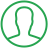# JavaScript Round To 1 Decimal

Last Updated : Jul 1, 2023In this tutorial we will show you the solution of JavaScript round to 1 decimal, here we defined decimal number with some amount of floating points we have to round those floating points and allow up to one digit only after point for that we used Math object.

Math object is a built-in object that provides properties and methods for mathematical constants functions to execute mathematical operations.

So we used Math.round() function to returns the value of a number rounded to the nearest integer.

## Step By Step Guide On JavaScript Round To 1 Decimal :-

Here we using round() method in Math object for gets the result. We defined random decimal number with floating points and stored to variable ‘num’ then using Number property EPSILON with 10 multiplication so we get one digits after floating point as a whole number.

The Math.round() method is rounds the last digit with nearest digit then we divided by 10 for sets floating point as one.

Finally we gets the result so we appends that with div element for displaying result in webpage.

```<!DOCTYPE html>
<html>
<title>ONE DECIMAL POINT</title>
<body>
<div id="res"></div>
<script>
var num=3726.748375;
num=Math.round((num+Number.EPSILON)*10)/10;
document.getElementById('res').innerHTML=num;
</script>
</body>
</html>```
1. <!DOCTYPE html> tag which is instruct the web browser about what version of HTML file written in and it’s not have any ending tag.
2. The<html> tag is used to indicate the beginning of HTML document.
3. As above shown <head> tag is contains information about webpage and external file links are declared here. <title> tag is used for set the webpage title.
4. Both <head> and <title> tags having their pair end tag, so we need to close the ending tags respectively. If you’re not closed anyone of ending tag properly that is also affect the webpage result.
5. <body> tag is beginning of main coding part because it contain coding of entire website blocks and elements described here.
6. In html we created <div> tag with ID ‘res’ for appends the result on webpage.
7. In <script> we defined variable namely ‘num’ with ‘var’ keyword and initialized value is ‘3726.748375’.
8. The Number with EPSILON property takes one digit after floating point to the left hand side and remaining digits presents after floating point by multiplication of 10.
9. Using Math.round() method after floating point presented digits removed and before floating point digit rounded as nearest digit then we gets floating point at before last one digit by dividing with 10.
10. Then the result is stored to variable ‘num’ and appends to html div element by ‘innerHTML’. So we appended result with div tag will displayed on webpage.
11. Both </body>, </html> tags closed respectively. </body> tag indicates the end of body, Then </html> tag indicates the end of HTML document.

## Conclusion :-

In conclusion now we are able to know how to round to one decimal place using javascript.

When we executing this program on browser we can see the result of floating with one digit number with concept heading displayed on webpage.

We can use toFixed() method also for getting same result but when we using round method we will get accurate round calculation result and we can set any number of floating digits by using this concept later we will discuss about this.

I hope this tutorial on JavaScript round to 1 decimal helps you and the steps and method mentioned above are easy to follow and implement.

##About Pragati

Experienced coding content writer who enjoys breaking down complex concepts in programming languages like Java, Python, C, and C++. Over three years of experience producing interesting and relevant content for a variety of entities. Committed to providing concise and easy-to-understand articles that assist readers in easily understanding technology and industry trends in the world of coding and software development.